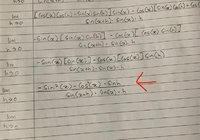Stuck on limits

Harry_the_cat

Elite Member
I think it should be the other way around. That is, the negative of what you have.

Jacob

New member
I think it should be the other way around. That is, the negative of what you have.
^
Could you please elaborate more on what you mean by that? I know that the answer is -csc^2x but I want to know the working so it would be easier for me to master it but I'm stuck on the part that I highlighted. This is my first time dealing with cosecant, cotangent & secant

Last edited:

Jacob

New member
I think it should be the other way around. That is, the negative of what you have.
In the lecture, my lecturer said that this 2 parts (which I marked x) will be canceled out. That makes sense but it didn’t when the next step he wrote the numerator as sinx sinx sinh - cosx cosh sinx.

Did I get it right?

Subhotosh Khan

Super Moderator
Staff member
Can someone please explain to me what’s happening to the numerator which I highlighted? It’s from my lecture but I don’t understand how it becomes sinx sinx sinh- cosx cosh sinx .
It does not! Do the algebra carefully!

Last edited:

Jacob

New member
In the lecture, my lecturer said that this 2 parts (which I marked x) will be canceled out. That makes sense but it didn’t when the next step he wrote the numerator as sinx sinx sinh - cosx cosh sinx.

Did I get it right?

It does not! Do the algebra carefully!
You mean this is incorrect?

Subhotosh Khan

Super Moderator
Staff member
In the lecture, my lecturer said that this 2 parts (which I marked x) will be canceled out. That makes sense but it didn’t when the next step he wrote the numerator as sinx sinx sinh - cosx cosh sinx.

Did I get it right?
No - I think it should be:

sin(x) * sin(x) * sin(h) - cos(x) * sin(h) * cos(x) ....,edited

Check it again......

Last edited:

Subhotosh Khan

Super Moderator
Staff member
This is exactly what my lecturer got. But I’m confused about how he got it. Why it becomes multiply? what happened to the negative sign in front of -sinx.

View attachment 24999
Sorry about the sloppy work !! Laying in bed and working with "kindle" is just my working. I have to get up and go to a real computer!!

•jonah2.0 and JeffM

Subhotosh Khan

Super Moderator
Staff member
just writing the numerator of your attachment (Original)

sin(x) * [cos(x)*cos(h) - sin(x)*sin(h)] - cos(x) * [sin(x)*cos(h) + cos(x)*sin(h)] ..................................multiply and distribute

sin(x) * [cos(x)*cos(h)] - sin(x) * [sin(x)*sin(h)] - cos(x) * [sin(x)*cos(h)] - cos(x)*[cos(x)*sin(h)] .................. cancel appropriate terms

sin(x) * [cos(x)*cos(h)] - sin(x) * [sin(x)*sin(h)] - cos(x) * [sin(x)*cos(h)] - cos(x)*[cos(x)*sin(h)]

= - sin(x) * [sin(x)*sin(h)] - cos(x)*[cos(x)*sin(h)]

= -sin(h)

A little later I'll delete my previous responses.

•Jacob

Jacob

New member
just writing the numerator of your attachment (Original)

sin(x) * [cos(x)*cos(h) - sin(x)*sin(h)] - cos(x) * [sin(x)*cos(h) + cos(x)*sin(h)] ..................................multiply and distribute

sin(x) * [cos(x)*cos(h)] - sin(x) * [sin(x)*sin(h)] - cos(x) * [sin(x)*cos(h)] - cos(x)*[cos(x)*sin(h)] .................. cancel appropriate terms

sin(x) * [cos(x)*cos(h)] - sin(x) * [sin(x)*sin(h)] - cos(x) * [sin(x)*cos(h)] - cos(x)*[cos(x)*sin(h)]

= - sin(x) * [sin(x)*sin(h)] - cos(x)*[cos(x)*sin(h)]

= -sin(h)

A little later I'll delete my previous responses.
Thank you. It totally make sense right now

Subhotosh Khan

Super Moderator
Staff member
Can someone please explain to me what’s happening to the numerator which I highlighted? It’s from my lecture but I don’t understand how it becomes sinx sinx sinh- cosx cosh sinx .
Now I hope you see that it is NOT sinx sinx sinh- cosx cosh sinx .........

•Jacob

jonah2.0

Full Member
Beer soaked ramblings follow.
Sorry about the sloppy work !! Laying in bed and working with "kindle" is just my working. I have to get up and go to a real computer!!
It's like I have a twin.

•topsquark

Subhotosh Khan

Super Moderator
Staff member
Beer soaked ramblings follow.

It's like I have a twin.
My Brother....

•topsquark

Jacob

New member
Now I hope you see that it is NOT sinx sinx sinh- cosx cosh sinx .........
Yes finally, thanks to you. May I know how you get

= - sin(x) * [sin(x)*sin(h)] - cos(x)*[cos(x)*sin(h)] (This part right here become -sin(h)?

= -sin(h)

Subhotosh Khan

Super Moderator
Staff member
Yes finally, thanks to you. May I know how you get

= - sin(x) * [sin(x)*sin(h)] - cos(x)*[cos(x)*sin(h)] (This part right here become -sin(h)?

= -sin(h)
- sin(x) * [sin(x)*sin(h)] - cos(x)*[cos(x)*sin(h)]

= -sin(x)*sin(x)*sin(h) - cos(x) *cos(x)*sin(h)

= -sin2(x) * sin(h) - cos2(x) * sin(h)

= - [sin2(x) + cos2(x)] * sin(h)

= -  * sin(h)

= - sin(h)

Please work with pencil and paper instead of staring at the screen!!

•Jacob

Jacob

New member
- sin(x) * [sin(x)*sin(h)] - cos(x)*[cos(x)*sin(h)]

= -sin(x)*sin(x)*sin(h) - cos(x) *cos(x)*sin(h)

= -sin2(x) * sin(h) - cos2(x) * sin(h)

= - [sin2(x) + cos2(x)] * sin(h)

= -  * sin(h)

= - sin(h)

Please work with pencil and paper instead of staring at the screen!!
Sorry, I did work through the problem. But this part = - [sin2(x) + cos2(x)] * sin(h) I got -ve instead +ve. May i know how it is +veHarry_the_cat

Elite Member
Have a closer look at Post #16. It IS negative.

•Jacob